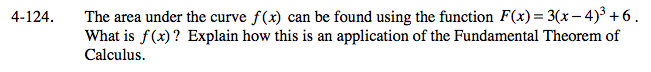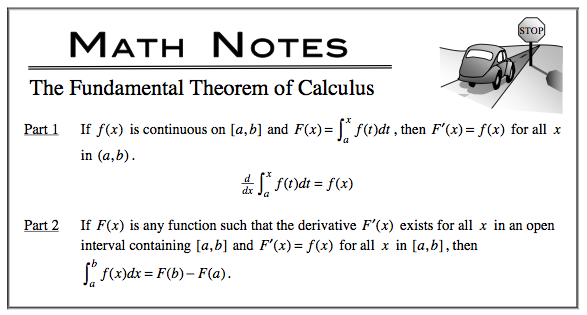### Home > CALC > Chapter 4 > Lesson 4.4.1 > Problem4-124

4-124.

The area under the curve f(x) can be found using the function F(x) = 3(x − 4)3 + 6 . What is f(x)? Explain how this is an application of the Fundamental Theorem of Calculus. Homework Help ✎Expand F(x) before antidifferentiating.